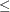### PRINT Statement

PRINT options ;

The PRINT statement is useful for displaying part of a solution summary, examining intermediate tableaus, performing sensitivity analysis, and using parametric programming. In the options, the colnames and rownames lists can be empty, in which case the LP procedure displays tables with all columns or rows, or both. If a column name or a row name has spaces or other special characters in it, the name must be enclosed in single or double quotes when it appears in the argument.

The options that can be used with this statement are as follows.

BEST

displays a Solution, Variable, and Constraint Summary for the best integer solution found.

COLUMN(colnames) / SENSITIVITY

displays a Variable Summary containing the logical and structural variables listed in the colnames list. If the / SENSITIVITY option is included, then sensitivity analysis is performed on the price coefficients for the listed colnames structural variables.

INTEGER

displays a Variable Summary containing only the integer variables.

INTEGER_NONZEROS

displays a Variable Summary containing only the integer variables with nonzero activity.

INTEGER_ZEROS

displays a Variable Summary containing only the integer variables with zero activity.

MATRIX(rownames,colnames) / PICTURE

displays the submatrix of the matrix of constraint coefficients defined by the rownames and colnames lists. If the / PICTURE option is included, then the formatted submatrix is displayed. The format used is summarized in Table 6.2.

Table 6.2: Format Summary

 Condition on the Coefficient x Symbols Printed abs(x) = 0 “ ” 0 < abs(x) < .000001 sgn(x) “Z” .000001abs(x) < .00001 sgn(x) “Y” .00001abs(x) < .0001 sgn(x) “X” .0001abs(x) < .001 sgn(x) “W” .001abs(x) < .01 sgn(x) “V” .01abs(x) < .1 sgn(x) “U” .1abs(x) < 1 sgn(x) “T” abs(x) = 1 sgn(x) “1” 1 < abs(x) < 10 sgn(x) “A” 10abs(x) < 100 sgn(x) “B” 100abs(x) < 1000 sgn(x) “C” 1000abs(x) < 10000 sgn(x) “D” 10000abs(x) < 100000 sgn(x) “E” 100000abs(x) < 1.0E06 sgn(x) “F”

NONINTEGER

displays a Variable Summary containing only the continuous variables.

NONINTEGER_NONZEROS

displays a Variable Summary containing only the continuous variables with nonzero activity.

NONZEROS

displays a Variable Summary containing only the variables with nonzero activity.

PRICESEN

displays the results of parametric programming for the current value of the PRICEPHI= option, the price coefficients, and all of the price change vectors.

RANGEPRICE

performs range analysis on the price coefficients.

RANGERHS

performs range analysis on the right-hand-side vector.

RHSSEN

displays the results of parametric programming for the current value of the RHSPHI= option, the right-hand-side coefficients, and all of the right-hand-side change vectors.

ROW(rownames) / SENSITIVITY

displays a constraint summary containing the rows listed in the rowname list. If the / SENSITIVITY option is included, then sensitivity analysis is performed on the right-hand-side coefficients for the listed rownames.

SOLUTION

displays the Solution Summary, including the Variable Summary and the Constraint Summary.

TABLEAU

displays the current tableau.

ZEROS

displays a Variable Summary containing only the variables with zero activity. This may be useful in the analysis of ON/OFF, ZERO/ONE, scheduling, and assignment applications.